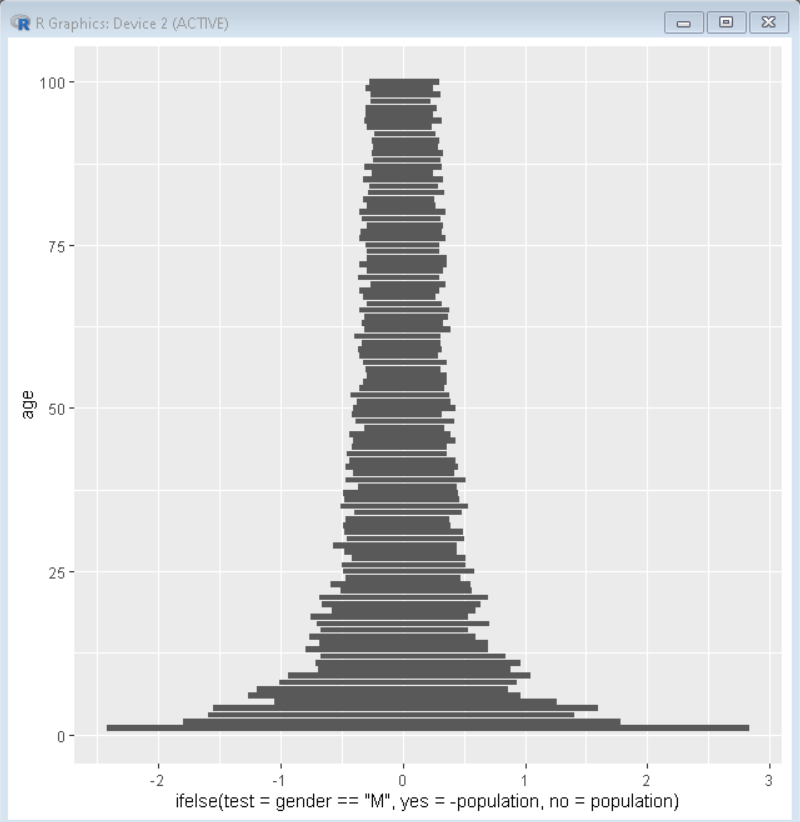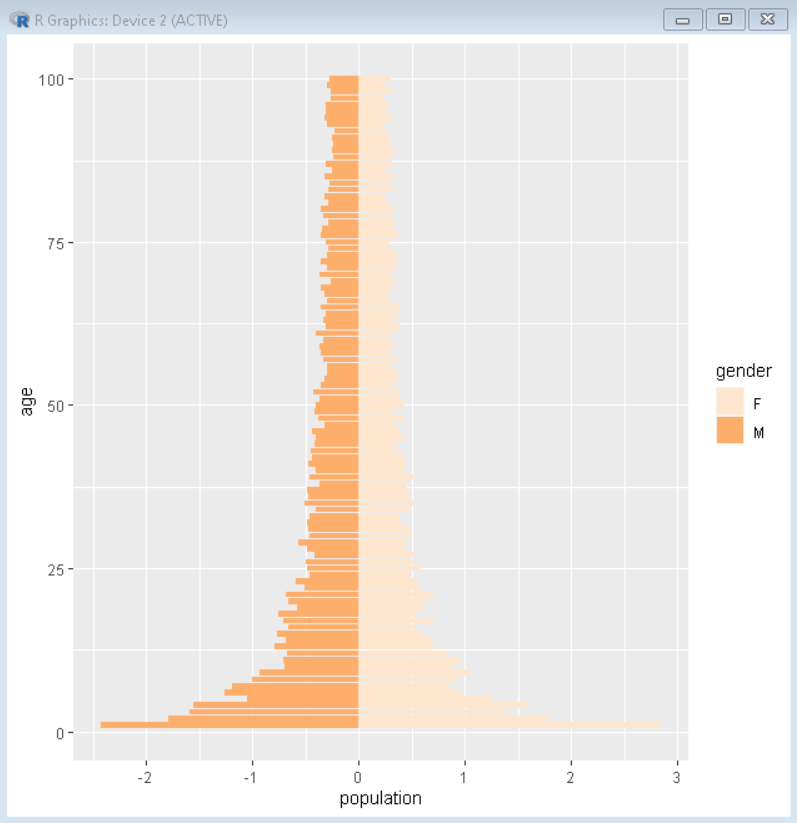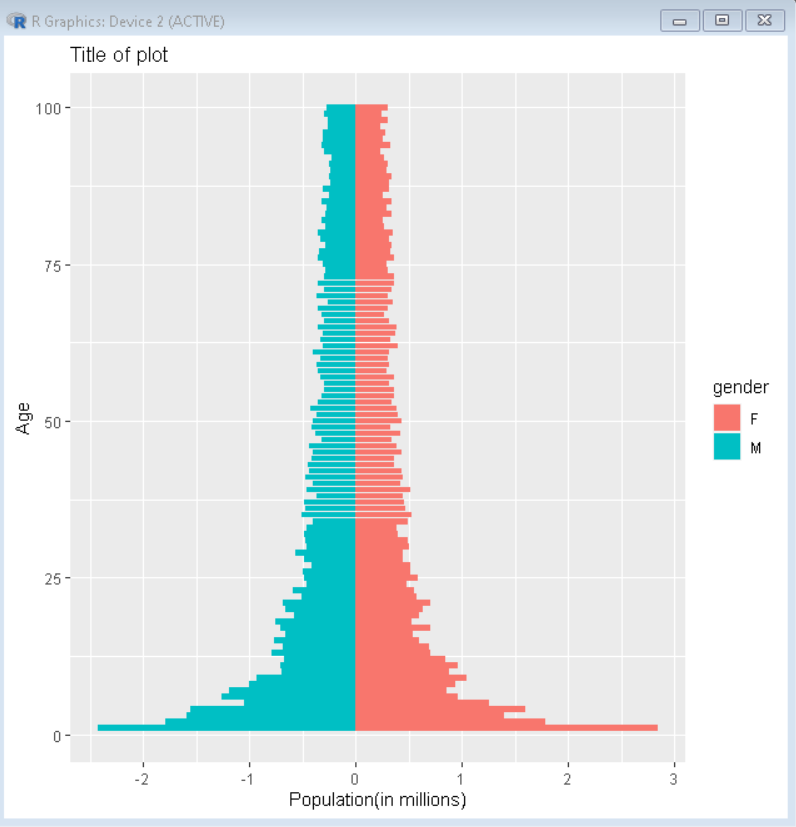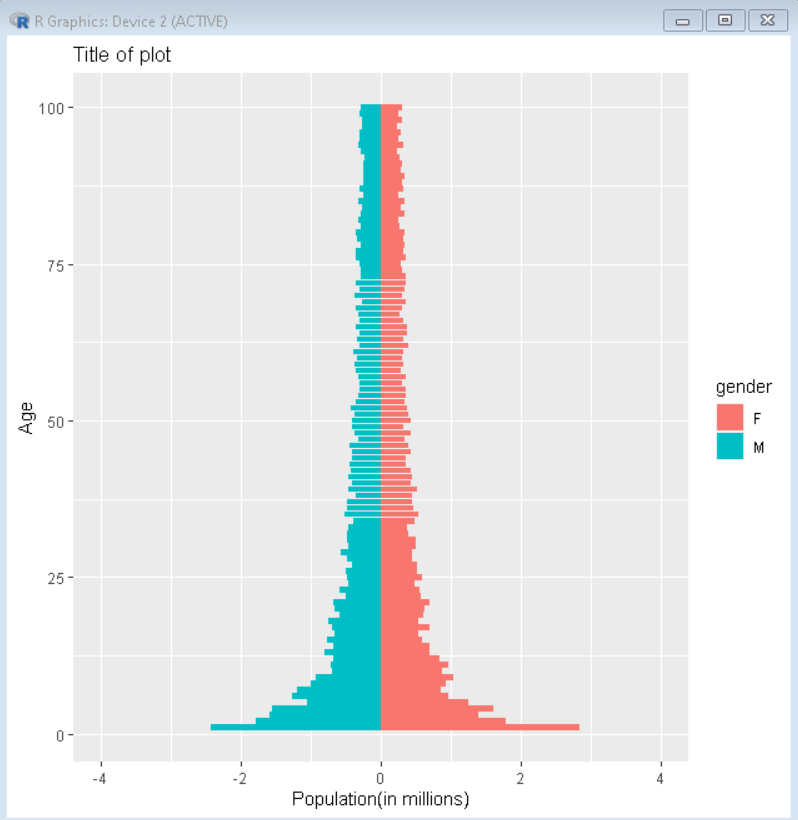# How to Create a Population Pyramid in R?

• Last Updated : 30 Jan, 2022

In this article, we will discuss how to create a population pyramid in the R Programming Language.

A population pyramid is also known as an age-sex pyramid. It helps us to visualize the distribution of a population by age group and sex. It generally takes the shape of a pyramid. In the population pyramid, males are usually depicted on the left and females on the right. This method of data visualization can be used to visualize the age of a particular population based on the number or percentage of male and female inhabitants.

To create a population pyramid in the R Language, we use the geom_bar() function of the ggplot2 package. The ggplot2 is a system for declaratively creating graphics, based on “The Grammar of Graphics”. The geom_bar() function is used to draw the bar plots, it makes the height of the bar proportional to the number of cases in each group.

## Basic creating a Population Pyramid in R

To create a population pyramid, we use the coord_flip() function along with the geom_bar() function to create a horizontal bar plot, then we make the value of the male population negative using the mutate function thus creating the male population bars on the left side and female population bar on the right side giving us the required population pyramid.

Syntax:

ggplot( df, aes(x = age, y = population)) +   geom_bar(stat = “identity”) + coord_flip()

Parameters:

• df: determines the data frame that contains population data.
• gender, age and population: determines the columns of df data frame.

Example:

Here, is a basic population pyramid. The CSV file used in the example can be downloaded here.

## R

 `# load sample data``sample_data <- ``read.csv``(``"Population.CSV"``)`` ` `# load library ggplot2 and dplyr``library``(ggplot2)``library``(dplyr)`` ` `# change male population to negative``sample_data %>%``mutate``(``    ``population = ``ifelse``(gender==``"M"``, population*(-1),``                        ``population*1))%>%``    ``ggplot``(``aes``(x = age,y = population)) + ``    ``geom_bar``(stat = ``"identity"``) +``    ``coord_flip``()`

Output:## Color Customization Population Pyramid in R

To customize the color of male and female bars, we use the fill aesthetic property of the ggplot() function. W can either pass the variable according to which we want the colors to be or we can pass the exact colors that need to be put. We can even use the scale_fill_brewer() function to set the color to a predefined palette.

Syntax:

ggplot( df, aes(x, y, fill) )

Parameters:

• fill: determines the variable according to which bars are to be colored.

Example:

Here, in this example wh have colored the plot in sequential palette number 7.

## R

 `# load sample data``sample_data <- ``read.csv``(``"Population.CSV"``)`` ` `# load library ggplot2 and dplyr``library``(ggplot2)``library``(dplyr)`` ` `# change male population to negative``sample_data %>%``mutate``(``    ``population = ``ifelse``(gender==``"M"``, population*(-1),``                        ``population*1))%>%``    ``ggplot``(``aes``(x = age,y = population, fill=gender)) + ``    ``geom_bar``(stat = ``"identity"``) +``    ``coord_flip``()+``    ``scale_fill_brewer``(type = ``"seq"``,palette = 7)`

Output:## Label Customization Population Pyramid in R

To customize the labels of the plot, we use the title, x, and y argument of the labs() function. Here, title, x, and y determine the title of the plot, the label of the x-axis, and the label of the y-axis respectively.

Syntax:

ggplot( df, aes(x, y) )+ labs( title, x, y)

Parameters:

• title: determines the title of the plot.
• x and y: determines the label of x and y axis respectively.

Example:

Here, is a population pyramid with custom colors and labels. The CSV file used in the example can be downloaded here.

## R

 `# load sample data``sample_data <- ``read.csv``(``"Population.CSV"``)`` ` `# load library ggplot2 and dplyr``library``(ggplot2)``library``(dplyr)`` ` `# change male population to negative``sample_data %>%``mutate``(``    ``population = ``ifelse``(gender==``"M"``, population*(-1),``                        ``population*1))%>%``    ``ggplot``(``aes``(x = age,y = population, fill=gender)) + ``    ``geom_bar``(stat = ``"identity"``) +``    ``coord_flip``()+``   ``labs``(title = ``"Title of plot"``, x = ``"Age"``, ``        ``y = ``"Population(in millions)"``)`

Output:### Axis Customization a Population Pyramid in R

Since in the above example, the population pyramid is not in the center because the population of females is larger. To solve these situations, we can fix the scale of the axis using the scale_x/y_continuous() function. We can also use this function to set the breaks in the axis. To set axis break, we use the breaks argument of the scale_x/y_continuous() function.

Syntax:

scale_x/y_continuous( limits, breaks)

Parameters:

• limits: determines the limits of the x or y-axis.
• breaks: determines the axis breaks of the x or y-axis.

Example:

Here, in this example, we have set y-axis limits to make the plot more uniform.

## R

 `# load sample data``sample_data <- ``read.csv``(``"Population.CSV"``)`` ` `# load library ggplot2 and dplyr``library``(ggplot2)``library``(dplyr)`` ` `# change male population to negative``sample_data %>%``mutate``(``    ``population = ``ifelse``(gender==``"M"``, population*(-1),``                        ``population*1))%>%``    ``ggplot``(``aes``(x = age,y = population, fill=gender)) + ``    ``geom_bar``(stat = ``"identity"``) +``    ``coord_flip``()+``    ``scale_y_continuous``(limits = ``c``(-4,4), ``                       ``breaks = ``seq``(-4, 4, by = 2))+``   ``labs``(title = ``"Title of plot"``, x = ``"Age"``,``        ``y = ``"Population(in millions)"``)`

Output:My Personal Notes arrow_drop_up